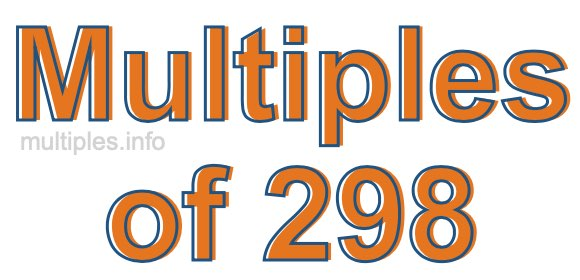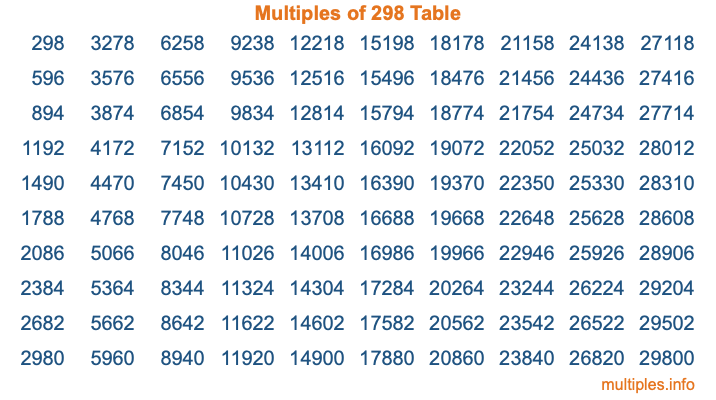Multiples of 298Welcome to the Multiples of 298 page. Here we will first teach you everything you will ever need to know about the multiples of 298, and then give you a study guide summary of everything we taught you to make sure you remember it all. Use this page to look up facts and learn information about the multiples of 298. This page will make you a multiples of two hundred ninety-eight expert!

Definition of Multiples of 298
Multiples of 298 are all the numbers that when divided by 298 equal an integer. Each of the multiples of 298 are called a multiple. A multiple of 298 is created by multiplying 298 by an integer.

Therefore, to create a list of multiples of 298, you start with 1 multiplied by 298, then 2 multiplied by 298, then 3 multiplied by 298, and so on for as long as you want. Thus, the list of the first five multiples of 298 is 298, 596, 894, 1192, and 1490. To see a larger list of multiples of 298, see the printable image of Multiples of 298 further down on this page. We also have a category where you can choose any nth multiple of 298.

Multiples of 298 Checker
The Multiples of 298 Checker below checks to see if any number of your choice is a multiple of 298. In other words, it checks to see if there is any number (integer) that when multiplied by 298 will equal your number. To do that, we divide your number by 298. If the the quotient is an integer, then your number is a multiple of 298.

Is  a multiple of 298?

Least Common Multiple of 298 and ...
A Least Common Multiple (LCM) is the lowest multiple that two or more numbers have in common. This is also called the smallest common multiple or lowest common multiple and is useful to know when you are adding our subtracting fractions. Enter one or more numbers below (298 is already entered) to find the LCM.

Check out our LCM Calculator if you need more details about the Least Common Multiple or if you need the LCM for different numbers for adding and subtraction fractions.

nth Multiple of 298
As we stated above, 298 is the first multiple of 298, 596 is the second multiple of 298, 894 is the third multiple of 298, and so on. Enter a number below to find the nth multiple of 298.

th multiple of 298

Multiples of 298 vs Factors of 298
298 is a multiple of 298 and a factor of 298, but that is where the similarities end. All postive multiples of 298 are 298 or greater than 298. All positive factors of 298 are 298 or less than 298.

Below is the beginning list of multiples of 298 and the factors of 298 so you can compare:

Multiples of 298: 298, 596, 894, 1192, 1490, etc.

Factors of 298: 1, 2, 149, 298

As you can see, the multiples of 298 are all the numbers that you can divide by 298 to get a whole number. The factors of 298, on the other hand, are all the whole numbers that you can multiply by another whole number to get 298.

It's also interesting to note that if a number (x) is a factor of 298, then 298 will also be a multiple of that number (x).

Multiples of 298 vs Divisors of 298
The divisors of 298 are all the integers that 298 can be divided by evenly. Below is a list of the divisors of 298.

Divisors of 298: 1, 2, 149, 298

The interesting thing to note here is that if you take any multiple of 298 and divide it by a divisor of 298, you will see that the quotient is an integer.

Multiples of 298 Table
Below is an image of the first 100 multiples of 298 in a table. The table is in chronological order, column by column. The first column has the first ten multiples of 298, the second column has the next ten multiples of 298, and so on.The Multiples of 298 Table is also referred to as the 298 Times Table or Times Table of 298. You are welcome to print out our table for your studies.

Negative Multiples of 298
Although not often discussed or needed in math, it is worth mentioning that you can make a list of negative multiples of 298 by multiplying 298 by -1, then by -2, then by -3, and so on, to get the following list of negative multiples of 298:

-298, -596, -894, -1192, -1490, etc.

Multiples of 298 Summary
Below is a summary of important Multiples of 298 facts that we have discussed on this page. To retain the knowledge on this page, we recommend that you read through the summary and explain to yourself or a study partner why they hold true.

There are an infinite number of multiples of 298.

A multiple of 298 divided by 298 will equal a whole number.

298 divided by a factor of 298 equals a divisor of 298.

The nth multiple of 298 is n times 298.

The largest factor of 298 is equal to the first positive multiple of 298.

298 is a multiple of every factor of 298.

298 is a multiple of 298.

A multiple of 298 divided by a divisor of 298 equals an integer.

298 divided by a divisor of 298 equals a factor of 298.

Any integer times 298 will equal a multiple of 298.

Multiples of a Number
Here you can get the multiples of another number, all with the same attention to detail as we did for multiples of 298 on this page.

Multiples of
Multiples of 299
Did you find our page about multiples of two hundred ninety-eight educational? Do you want more knowledge? Check out the multiples of the next number on our list!